# Fastai v2 code walk-thru 2

Notes for this walk-thru from @Honigtoast:

# Notebook 10 Pets Tutorial

Hint: Always make sure to git pull before you start!
The Notebook can be found here: Notebook 10. And here is the lesson video.

## Pipelines:

Q: Can we decode from Pipelines?

A: Similar to encoding, we can also use Pipelines to decode! If you are using a pipeline to decode something, it will pipe through all the steps and call `decode` on each.

`pipe.show` is an interesting pipeline: when you want to show a pipeline, it is going to decode every part of the tuple. It will stop as soon as it gets to a type which has a `show` method defined.

Example:

`t` is a tuple of a `PILImage` and a number.
This decodes each part of the tuple by decoding each of the pipelines.
It ends up with the name of the dog breed.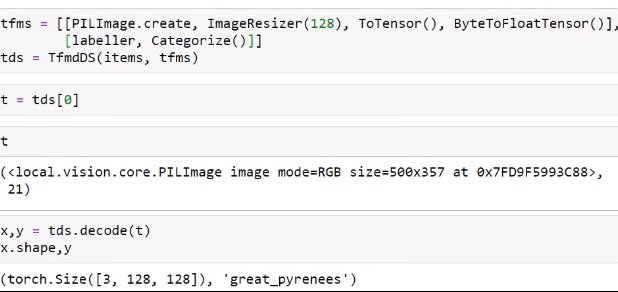`TfmDL` understands two things:

1. How to maintain types (makes sure PILImage and subtypes are maintained);
2. Decoding (you can have transforms in a TfmDL and they behave the same way as in a TfmDS).

### Compared to last time:

• The labeller pipeline is still the same
• But: the Image pipeline is just PILImage.create
• class PILImage(PILBase): pass
• PILBase has a create-method and a show-method

### Why are we able to get rid of these three things in our pipeline?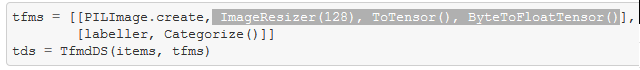-> We moved them into our DataLoader pipeline

## DataSource

`DataSource` is basically a transformed dataset `TfmDS` with the ability to create different training sets and validation sets (in fact as many subsets as you like).

Additionally to the things we passed in to `TfmDS`, we will pass the indices of the splits into the `DataSource`:

``````pets = DataSource(items, tfms, filts=split_idx)
``````

`len(split_idx)` has two arrays in it which are just a list of Ids for train/valid set.

You can use the same functionality as with `TfmDS`, but you can also ask for a subset:`x,y = pets.subset(1)` This is the validation set which has the shortcut `pets.valid`

`pets.subset(0)` is the training set with the shortcut `pets.train`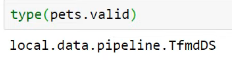You can do everything with this just like before with `TfmDS`!

### Special pipelines:

`after_item=ds_img_tfms` transform that runs on each individual tuple

`after_batch=dl_tfms` runs after the tuples have been collated together by the PyTorch `DataLoader` into a single batch. This means we are able to easily run GPU-transforms which will be much faster than on CPU!

### What happens, when a pipeline first gets its DataSource?

Example with `Categorize()`

• It first calls the special method `setup()`
• If there is a training-subset of the `DataSource`, it will be grabbed here
• This ensures that your category-vocab is only done on the training-set

## Segmentation

``````cv_source = untar_data(URLs.CAMVID_TINY)
cv_items = get_image_files(cv_source/'images')
cv_splitter = RandomSplitter(seed=42)
cv_split = cv_splitter(cv_items)
cv_label = lambda o: cv_source/'labels'/f'{o.stem}_P{o.suffix}'
``````
1. Grab a subset of CAMVID
2. Get the image files
3. Create a RandomSplitter
4. Create the split indexes
5. Create a labeling function
``````tfms = [[PILImage.create], [cv_label, PILMask.create]]
camvid = DataSource(cv_items, tfms, filts=cv_split)
trn_dl = TfmdDL(camvid.train,  bs=4, after_item=ds_img_tfms, after_batch=dl_tfms)
- Transforms are basically the same as we have seen before. But: for y we first call cv_label which grabs the name of the mask-file from the image-file
- class PILMask(PILBase): _open_args,_show_args = {'mode':'L'},{'alpha':0.5, 'cmap':'tab20'}
- Then we call PILMask.create which is just a single line of code. With this we can do seperate encodes for PILImages and PILMasks (doing different functionality for different types).
``````

Note: This style of intermediate API is chosen for the purpose of being very transparent and easy to use. (compared to the higher DataBlocks API)

# Notebook 06 Data Transforms

The Notebook can be found here: Notebook 06

### What we will learn:

We will take a look into fastai.data.blocks with the tiny MNIST dataset (which only consists of 3s and 7s). This is not a tutorial notebook! This notebook actually defines data.core and ends up with defining /local/data.py

• How `get_files` and `get_image_files` work
• Splitter
• Labeller
• Categorize and Multicategorize
• MNIST example
• TfmDL

## getting items

``````def get_files(path, extensions=None, recurse=True, include=None)
``````

Basic `get` is just as in fastai v1: `get_files` takes 4 parameters

• `Path` : where we are looking for files in
• `extensions`: which file extensions to look for
• `recurse`: if we want to do this recursively
• `include`: is there a subset to include

### /local/data.py

Automaticall created `__all__`: this is used by python to decide what will be imported if you say `import *`

### back to get

An item can be kind of anything you like:
- For vision, it is normally a list of paths
- An item list can also be a `DataFrame`, connection to a `DataBase`, a network, a pipe… etc
- For now we are using these with paths and are looking at DataFrames later.

``````# export
def _get_files(p, fs, extensions=None):
p = Path(p)
res = [p/f for f in fs if not f.startswith('.')
and ((not extensions) or f'.{f.split(".")[-1].lower()}' in extensions)]
return res
``````
``````# export
def get_files(path, extensions=None, recurse=True, include=None):
"Get all the files in `path` with optional `extensions`, optionally with `recurse`."
path = Path(path)
extensions = setify(extensions)
extensions = {e.lower() for e in extensions}
if recurse:
res = []
for i,(p,d,f) in enumerate(os.walk(path)): # returns (dirpath, dirnames, filenames)
if include is not None and i==0: d[:] = [o for o in d if o in include]
else:                            d[:] = [o for o in d if not o.startswith('.')]
res += _get_files(p, f, extensions)
else:
f = [o.name for o in os.scandir(path) if o.is_file()]
res = _get_files(path, f, extensions)
return L(res)
``````

### tests in the documentation

Used to ensure functionality.

``````t3 = get_files(path/'train'/'3', extensions='.png', recurse=False)
t7 = get_files(path/'train'/'7', extensions='.png', recurse=False)
t = get_files(path/'train', extensions='.png', recurse=True)
test_eq(len(t), len(t3)+len(t7))
test_eq(len(get_files(path/'train'/'3', extensions='.jpg', recurse=False)),0)
test_eq(len(t), len(get_files(path, extensions='.png', recurse=True, include='train')))
t
``````

Sometimes there are additional checks that are not that interesting for documentation purposes but just useful as tests. These are marked with "#hide" so that it will not be included in the docs.

The docs are here: fastai v2 docs

Sometimes there are CamelCase functions that return functions, like `FileGetter`, and this is similar to partial functions in Python.

### get_image_files

``````def get_image_files(path, recurse=True, include=None):
"Get image files in `path` recursively."
return get_files(path, extensions=image_extensions, recurse=recurse, include=include)
``````

This is just calling `get_files` with the additional parameter for `image_extensions` (this is everything within mimetypes which starts with ‘image’)

## Splitting things

The functions return two lists for training and validation sets. The lists can contain either indices or masks.

Splitting things randomly with `RandomSplitter` where you optionally can pick a specific seed.

``````def RandomSplitter(valid_pct=0.2, seed=None, **kwargs):
"Create function that splits `items` between train/val with `valid_pct` randomly."
def _inner(o, **kwargs):
if seed is not None: torch.manual_seed(seed)
rand_idx = L(int(i) for i in torch.randperm(len(o)))
cut = int(valid_pct * len(o))
return rand_idx[cut:],rand_idx[:cut]
return _inner
``````

With GrandparentSplitter you can look at the parent of the parent. In MNIST oder IMAGENET that would be the path for valid or train.

Note: More documentation will be added. You can also contribute to the docs if you are interested!

## Labeling things

For labeling you need something that takes a `Path` and gets a label out of it.

``````def parent_label(o, **kwargs):
"Label `item` with the parent folder name"
return o.parent.name if isinstance(o, Path) else o.split(os.path.sep)[-1]
``````

`parent_label` labels everything with the name of the parent. Nothing to customize in this case, so no returned function and therefore no capital letters for the functions name.

``````def RegexLabeller(pat):
"Label `item` with regex `pat`."
pat = re.compile(pat)
def _inner(o, **kwargs):
res = pat.search(str(o))
assert res,f'Failed to find "{pat}" in "{o}"'
return res.group(1)
return _inner
``````

`RegexLabeller` is again CamelCase. We need to pass in a pattern for what we are regex labelling things with.
Note: if you want to understand how stuff works, just open these notebooks like in the fastai lessons and experiment with them!

# Categorize

`Categorize` is a `Transform` and so it has `encodes` and `decodes`. When you create a `Categorize` object, the dunder init function just works in the usual way. It can be created with a predefined vocab which will be assigned within `__init__`, but if not, the special `setup` method is used.

``````# export
class Categorize(Transform):
"Reversible transform of category string to `vocab` id"
order=1

def setup(self, dsrc):

def encodes(self, o): return self.vocab.o2i[o]
def decodes(self, o)->Category: return self.vocab[o]
``````
``````cat = Category.create()
tds = TfmdDS(['cat', 'dog', 'cat'], tfms=[cat])
``````
• `['cat', 'dog', 'cat']` list of items
• `tfms=[cat]` list of transforms
• `TfmDS` is creating a number of different lists `TfmdList`
• `TfmdList` will call `self.setup` in the `__init__`
• `self.setup` will setup your transforms which again calls setup in the `Categorize`
``````def setup(self, dsrc):
``````

This sets the vocab to CategoryMap which uses the train-datasource if it has a train attribute and otherwise will just use the whole dsrc.

``````class Category(str, ShowTitle): _show_args = {'label': 'category'}
``````

`decodes` returns `Category` which is a tiny class that inherits from `ShowTitle` and this simply has a show-method.

``````class CategoryMap(CollBase):
if is_categorical_dtype(col): items = L(col.cat.categories)
else:
# `o==o` is the generalized definition of non-NaN used by Pandas
items = L(o for o in L(col).unique() if o==o)
if sort: items = items.sorted()
self.items = '#na#' + items if add_na else items
self.o2i = defaultdict(int, self.items.val2idx())
def __eq__(self,b): return all_equal(b,self)
``````

`CategoryMap` grabs all of the unique values in your column, optionally sort them, and then optionally creates the object-to-int `o2i`.

## Multicategorize

Like in the planet-dataset, this is used for when each item can have mutiple labels.

Here, every item has a list as labels. Item number 1 is labeled with b and c, item number 2 is labeled with a and item number 3 is labeled with a and c.

``````cat = MultiCategorize()
tds = TfmdDS([['b', 'c'], ['a'], ['a', 'c']], tfms=[cat])
test_eq(cat.vocab, ['a', 'b', 'c'])
test_eq(cat(['a', 'c']), [0,2])
``````

Tests: The vocab should be a,b,c and categorizing a and c should give us 0 and 2.

``````# export
class MultiCategorize(Categorize):
"Reversible transform of multi-category strings to `vocab` id"
def setup(self, dsrc):
if not dsrc: return
if self.vocab is None:
dsrc1 = getattr(dsrc,'train',dsrc)
vals = set()
for b in dsrc1: vals = vals.union(set(b))
self.vocab,self.o2i = uniqueify(list(vals), sort=True, bidir=True)
setattr(dsrc, 'vocab', self.vocab)

def encodes(self, o):                return [self.o2i  [o_] for o_ in o]
def decodes(self, o)->MultiCategory: return [self.vocab[o_] for o_ in o]
``````

This is very similar as before, only that `encodes` and `decodes` have to do it for everything in the list.
`show` method for `MultiCategory` is a bit different as well because we have multiple things to show. Therefore we use this string joint in python `sep.join(self.mapped(str)`

``````class MultiCategory(L):
def show(self, ctx=None, sep=';', **kwargs): return show_title(sep.join(self.mapped(str)), ctx=ctx)
``````

### test_stdout

``````test_stdout(lambda: tds.show_at(2), 'a;c')
``````

We will learn about this more later, but this is a super handy test function. The `show` method actually prints something and the problem here is: how do you test if something is printed correctly? So, this function will actually test if `tds.show_at(2)` prints out `'a;c'` or not.

### One-hot encoding

Usually, multi category stuff needs to be one-hot encoded. This works the same way as in fastai v1.

``````# export core
def one_hot(x, c):
"One-hot encode `x` with `c` classes."
res = torch.zeros(c, dtype=torch.uint8)
res[L(x)] = 1.
return res
``````

This is done with this Transform:

``````# export
class OneHotEncode(Transform):
"One-hot encodes targets and optionally decodes with `vocab`"
order=2
def __init__(self, do_encode=True, vocab=None): self.do_encode,self.vocab = do_encode,vocab

def setup(self, dsrc):
if self.vocab is not None: self.c = len(self.vocab)
else: self.c = len(L(getattr(dsrc, 'vocab', None)))
if not self.c: warn("Couldn't infer the number of classes, please pass a `vocab` at init")

def encodes(self, o): return one_hot(o, self.c) if self.do_encode else tensor(o).byte()
def decodes(self, o): return one_hot_decode(o, self.vocab)
``````
• `encodes` calls `one_hot`
• `decodes` calls `one_hot_decode`

Mini Test: taking a bunch of items and passing it through a pipeline (which contains `MultiCategorize` and `OneHotEncode`).

``````tds = TfmdDS([['b', 'c'], ['a'], ['a', 'c']], [[MultiCategorize(), OneHotEncode()]])
test_eq(tds, [tensor([1, 0, 0]).byte()])
test_eq(tds.decode([tensor([0,1,1])]), [['b','c']])
test_stdout(lambda: tds.show_at(2), 'a;c')
``````

## Putting it all together for MNIST

Getting the image files:

``````items = get_image_files(path)
splits = splitter(items)
train,valid = (items[i] for i in splits)
train[:3],valid[:3]
``````

We have not created anything from the vision application yet, so we would not want to `#export` anything with Pillow, but we can use them as tests.

``````from PIL import Image
def open_img(fn:Path)->Image.Image: return Image.open(fn).copy()
def img2tensor(im:Image.Image)->TensorImage: return array(im)[None]
``````

Here are two transform pipelines and the `TfmdDS` which takes the training and the testset:

``````tfms = [[open_img, img2tensor],
[parent_label, Categorize()]]
train_ds = TfmdDS(train, tfms)

``````

Then, we grab one item of it, decode it, and test it.

``````x,y = train_ds
xd,yd = train_ds.decode_at(3)
test_eq(parent_label(train),yd)
test_eq(array(Image.open(train)),xd)
``````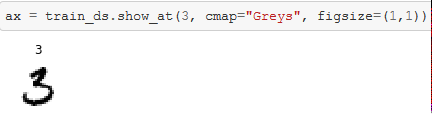`show_at` takes the `TfmdDS` and shows it at point 3 with optional parameters that are passed along to matplotlib.

### img2tensor

Q: img2tensor is actually converting image into numpy array not torch array, is this a desired approach?

A: The return type here is `TensorImage` and this is only calling `pass`. This is just used to mark the type so that transforms can be created that are restricted to that type. But basically this is just a subclass of Tensor (the numpy array is cast to tensor). And actually this is not a transform, but a function. The thing is that everything that is not a transform (but just a function) will be turned into a transform when you create something that is turned into a pipeline.

``````from PIL import Image
def open_img(fn:Path)->Image.Image: return Image.open(fn).copy()
def img2tensor(im:Image.Image)->TensorImage: return array(im)[None]
``````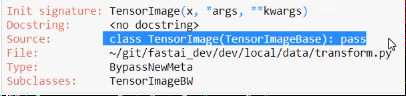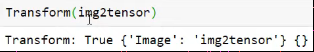This creates a transform object that will run that function.

## TfmdDL

`TfmdDL` inherits from `DataLoader` which is not a PyTorch DataLoader, but a fastai `local.data.load.DataLoader` which has extra stuff added to Pytorches DataLoader. `TfmdDL` turns this DataLoader into something that can understand transforms. For a full understanding, we need to look at the code for `DataLoader` which will be done next time.

``````tfms = [[open_img], [parent_label, Categorize()]]
tds = TfmdDS(items, tfms)
tdl = TfmdDL(tds, after_item=img2tensor, bs=4)
b = tdl.one_batch()
test_eq(L(tdl.decode_batch(b)).mapped(type), (TensorImage,Category))
``````

Some familiar transforms: creating a Pillow Image and label the categories. `TfmdDL` then takes the dataset `tds` and converts the images to tensors in the DataLoader. Here, the batchsize is 4 (4 images and 4 labels).

`test_eq(L(tdl.decode_batch(b)).mapped(type), (TensorImage,Category))` tests if the decode version of the batch when we map the function `type` over it is TensorImage,Category. This is interesting because it shows that fastai knows hot to take a tensor that comes out of the DataLoader (and which does not generally have types) and will put the correct types back onto it. Handy for inference so that in production you are going to have proper types!

Going through this line of code in detail: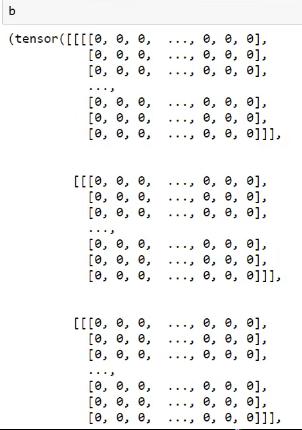Decoded version of b:Rather than 2 things we now have got 4 things: 4 pairs of image and label.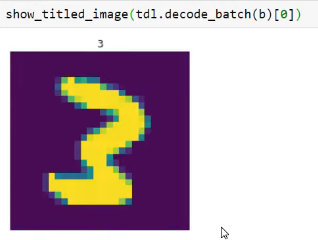This output is a bit awkward: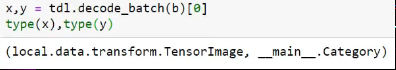So we wrap this in an `L` and map the function `type` over it: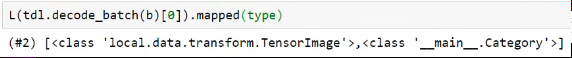`TfmdDL` has a one_batch method which actually is from DataLoader.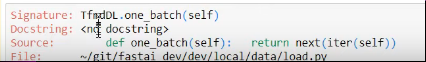### NegTfm

`train_ds` is an image and a label and the type of that is `local.data.transform.TensorImage`.

``````class NegTfm(Transform):
def encodes(self, x): return -x
def decodes(self, x): return -x
tdl = TfmdDL(train_ds, after_batch=NegTfm(), bs=4, num_workers=4)
b = next(iter(tdl))
test_eq(type(b), TensorImage)
``````

Here we check if the type is still `TensorImage` after going through the transform.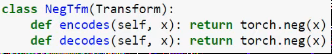These still pass the test, either though it looks like they should not pass! The reason is, that all of the transformation pipelines check (after it goes through `encodes`and `decodes`) that the type does not change. And if it does change, it will convert the type back to what it used to be, but only in special situations like here: you end up with a superclass `torch.Tensor` of the input (whereas the input was a subclass of that).

## Back to TfmdDL

### Decorator @delegates()

``````#export
@delegates()
def __init__(self, dataset, bs=16, shuffle=False, num_workers=None, **kwargs):
if num_workers is None: num_workers = min(16, defaults.cpus)
for nm in _dl_tfms:
kwargs[nm] = Pipeline(kwargs.get(nm,None), as_item=(nm=='before_batch'))
kwargs[nm].setup(self)
super().__init__(dataset, bs=bs, shuffle=shuffle, num_workers=num_workers, **kwargs)
it  = self.do_item(0)
its = self.do_batch([it])
#TODO do we still need?
self._retain_ds = partial(retain_types, typs=L(it ).mapped(type))
self._retain_dl = partial(retain_types, typs=L(its).mapped(type))
``````

The interesting thing here is the decorator `@delegates()`. Jeremy wrote an interesting article about delegation: Make Delegation Work in Python
Quick summary of what’s going on:

• `TfmdDL` is a subclass of `DataLoader`
• When calling `__init__` it wants to pass along any keyword arguments `**kwargs` that you passed in. Normally, there are a couple of issues when putting `**kwargs` like this:
• When we want to use Shift+Tab: rather than seeing the list of things we can pass in, we will see `**kwargs`. But this is not what happens here, you can see the actual arguments and use the code completion for it!

### show_batch

``````def show_batch(self, b=None, max_n=10, ctxs=None, **kwargs):
"Show `b` (defaults to `one_batch`), a list of lists of pipeline outputs (i.e. output of a `DataLoader`)"
if b is None: b = self.one_batch()
b = self.decode(b)
if ctxs is None:
if hasattr(b, 'get_ctxs'): ctxs = b.get_ctxs(max_n=max_n, **kwargs)
else: ctxs = [None] * len(b if is_iter(b) else b)
db = self._decode_batch(b, max_n, False)
ctxs = [self.dataset.show(o, ctx=ctx, **kwargs) for o,ctx in zip(db, ctxs)]
if hasattr(b, 'display'): b.display(ctxs)
``````

We saw `show_batch` in the segmentation and pets section like this:

The idea is: it takes an input and knows how to display it according to the input. Like in pets, it shows the images and the categories, or in the segmentation, it shows a mask and an image.
Things that can be passed in:

• A batch to show (or default is one batch)
• Contexts: a grid of plots. Each one of the above will be a different `ctxs`
6 Likes

Notes for code walk-thru 2 (Still working - Not complete)

## What was covered in the last lesson?

1. Created a PetTfm class which derives from Transform and use it as a function. Transform would pass the arguments to the encode function like how PyTorch nn.Module passes it to the forward method. encodes function would resize the image and create a label. It optionally contains decode function which helps to undo and helps in displaying it.
``````class PetTfm(Transform):
def __init__(self, items, train_idx):
self.items,self.train_idx = items,train_idx
self.labeller = RegexLabeller(pat = r'/([^/]+)_\d+.jpg\$')
vals = map(self.labeller, items[train_idx])
self.vocab,self.o2i = uniqueify(vals, sort=True, bidir=True)

def encodes(self, i):
o = self.items[i]
return resized_image(o), self.o2i[self.labeller(o)]

def decodes(self, x)->TitledImage: return x,self.vocab[x]
``````
1. Pipeline functions which help in composing functions together or transforms. When one of the transformations returns a tuple of elements then pipeline would apply the transformation to the elements of the tuple.
``````pipe = Pipeline([sp, OpenAndResize(), SiameseImage.create])
``````
1. TfmDS takes an arrays of transforms and converts each one of them to a pipeline. It then applies the pipeline to each element of the item list.
``````tfms = [[PILImage.create, ImageResizer(128), ToTensor(), ByteToFloatTensor()],
[labeller, Categorize()]]
tds = TfmdDS(items, tfms)
``````

## Topics covered today

In today’s lesson, Jeremy starts with TfmDL. TfmDL is a data loader which understands fastai v2 specifics like how to maintain types. For example, it ensures transforms or PILImage subtypes change and understand decoding. You can pass transforms to a TfmDL which behaves similar to TfmDS. Lets create a TfmDL.

``````tfms = [[PILImage.create], [labeller, Categorize()]]
tds = TfmdDS(items, tfms)
tdl = TfmdDL(tds, bs=1, after_item=[ImageResizer(128), ToTensor(), ByteToFloatTensor()])
``````

The `tfms` contains transformations for Image(tfms) and the lables(tfms). The image transformations contains only create and the rest of the transformations are moved to data loader transformations using after_item. Transformations in after_item are called once the dataset is created. One important thing to note is the ImageResizer,ByteToFloatTensor transformations does not make sense to be applied to Categorize. ImageResizer transformation is applied only to the images as the encode methods are defined only for PILImage and PILMask.

``````class ImageResizer(Transform):
order=10
"Resize image to `size` using `resample"
def __init__(self, size, resample=Image.BILINEAR):
if not is_listy(size): size=(size,size)
self.size,self.resample = (size,size),resample

def encodes(self, o:PILImage): return o.resize(size=self.size, resample=self.resample)
def encodes(self, o:PILMask):  return o.resize(size=self.size, resample=Image.NEAREST)
``````

ByteToFloatTensor also behaves similarly as its encode methods are defined for TensorImage and TensorMask.

``````class ByteToFloatTensor(Transform):
"Transform image to float tensor, optionally dividing by 255 (e.g. for images)."
order = 20 #Need to run after CUDA if on the GPU
def __init__(self, div=True, div_mask=False, filt=None, as_item=True):
super().__init__(filt=filt,as_item=as_item)

def encodes(self, o:TensorImage): return o.float().div_(255.) if self.div else o.float()
def decodes(self, o:TensorImage): return o.clamp(0., 1.) if self.div else o
``````

### What is PILImage

PILImage is a class which inherits from PILBase.

``````class PILImage(PILBase): pass
``````

### What is PILBase

PILBase inherits from Image.Image and contains 2 factory methods create for loading the image which takes a file name and show which displays the image which is used in the pipeline.

``````class PILBase(Image.Image, metaclass=BypassNewMeta):
default_dl_tfms = ByteToFloatTensor
_show_args = {'cmap':'viridis'}
_open_args = {'mode': 'RGB'}
@classmethod
def create(cls, fn, **kwargs)->None:
"Open an `Image` from path `fn`"

def show(self, ctx=None, **kwargs):
"Show image using `merge(self._show_args, kwargs)`"
return show_image(self, ctx=ctx, **merge(self._show_args, kwargs))
``````

### DataSource

DataSource is a transformed dataset with the ability to create train and validation sets. It is also flexible to allow to create as many datasets as required. DataSource behaves similar to TfmDS except, it takes a list of indexes for the subsets.

#### Example

``````tds = TfmdDS(items, tfms)
pets = DataSource(items, tfms, filts=split_idx)

x,y = pets.subset(1)
x.shape,y
OutPut : ((500, 362), 10)

#Alternative method
x2,y2 = pets.valid
``````

Each subset of the DataSource is of type TfmDS and we can apply things like decode, show to the subsets.

Another important part of DataSource is transformations that can be applied after batching. It provides benefits like running in the GPU. An obvious batch transformation is moving a batch of data to Cuda. We also do ByteToFloatTensor on GPU, it provides various benefits like lesser storage to move to GPU and the computation also takes nontrivial time on the CPU.

#### Example

``````ds_img_tfms = [ImageResizer(128), ToTensor()]
dl_tfms = [Cuda(), ByteToFloatTensor()]

trn_dl = TfmdDL(pets.train, bs=9, after_item=ds_img_tfms, after_batch=dl_tfms)
b = trn_dl.one_batch()
``````

It is important to notice that we are not doing anything special for moving the computations to GPU. Let’s look at the encode function of ByteToFloatTensor.

``````def encodes(self, o:TensorImage): return o.float().div_(255.) if self.div else o.float()
``````

As we can see from the example that it is tensor computations and broadcasting. We are not writing anything differently.

We can use show_batch on `trn_dl` to see a set of images.

#### Q&A

Does ByteToFloatTensor Normalize ?
It does not. It converts data to a range of 0 to 1. There is a separate `Normalize` transformation which does normalize.

Can Text transformation be done in GPU?
No, most of the text transformation is done in the preprocessing stage once. Text transformations are very fast nowadays.

Why we do not do all transformations in GPU?
There are many reasons. In order to process efficiently in GPU, we need batches and all the images in the batches should be of the same size. Some of the basic transformations you may want to do in the CPU are loading an image, resizing it and converting to Tensor. Nvidia dali may be an alternative as they are building custom Cuda kernels which can handle images of different sizes. But it is in its early stages.

Do images need to be rectangular?
Definitely not, we will look at more examples later.

What kind of transformations are recommended to do in GPU vs CPU?
Try to do everything in GPU except for resizing images.

#### Example

Let’s look at how to create a TfmDL for a segmentation task.

``````cv_source = untar_data(URLs.CAMVID_TINY)
cv_items = get_image_files(cv_source/'images')
cv_splitter = RandomSplitter(seed=42)
cv_split = cv_splitter(cv_items)
cv_label = lambda o: cv_source/'labels'/f'{o.stem}_P{o.suffix}'

camvid = DataSource(cv_items, tfms, filts=cv_split)
trn_dl = TfmdDL(camvid.train,  bs=4, after_item=ds_img_tfms, after_batch=dl_tfms)
``````

As we can see the steps(Download datasets, get file names, create train,validation splits, creare transformations, DataSource and DataLoader) is pretty similar to how we did it for the above classification task. The key difference is the functions applied for the labels. cv_label function is used to get label from the file name and PILMask is used to indicate the ImageResizer to use a different resample function.

``````class ImageResizer(Transform):
order=10
"Resize image to `size` using `resample"
def __init__(self, size, resample=Image.BILINEAR):
if not is_listy(size): size=(size,size)
self.size,self.resample = (size,size),resample

def encodes(self, o:PILImage): return o.resize(size=self.size, resample=self.resample)
def encodes(self, o:PILMask):  return o.resize(size=self.size, resample=Image.NEAREST)
``````

We can also observe that transforms like `ds_img_tfms` and `dl_tfms` are same as the ones used for pets data loader. The idea of intermediate block APIs between the data block API’s and lowest level is to make customizing super easy. Which is very essential for people doing kaggle and doing research.

#### Q&A

What is the best way to profile fastai code to see GPU / CPU / io bottlenecks? Is there a way to do that in one place?
Jeremy generally uses `htop` to see CPU utilization and `nvidia-smi dmon` for GPU utilization. The key column to look at while checking for GPU utilization is sm, it should be around 90 to 100% during training. If it is less than it means GPU is being underutilized and CPU’s are being overtaxed. If CPU’s are being overutilized then you can use python profilers to understand why.

## data.core

We have looked at a tutorial notebook `08_pets_tutorial.ipynb` till now. We will be looking into `05_data_core.ipynb` notebook which defines the module called data.core in the library.

The first few lines of the module defines the classes and the functionalities that needs to be imported.

``````__all__ = ['get_files', 'FileGetter', 'image_extensions', 'get_image_files', 'ImageGetter', 'RandomSplitter',
'GrandparentSplitter', 'parent_label', 'RegexLabeller', 'CategoryMap', 'Category', 'Categorize',
'MultiCategory', 'MultiCategorize', 'one_hot_decode', 'OneHotEncode', 'ToTensor', 'TfmdDL', 'Cuda',
``````

When we specify `from local.core.module import *` python imports only the said functions and classes. In most of the python libraries it is not declared and as a result everything gets imported in a nested fashion. fastai takes care to only include the essentials to avoid such issues. As these lines are automatically created we would only see it in the python modules and not in the notebook.

This is our first deep dive into literate programming as we are building data.core module with pros along the way.

data.core contains some of the helper functions to process data.

Get, split and label.

### Get

get_files Given a path to a folder it can scan for all the files recursively and extract files with particular extensions. We can also customize the functionality by specifying what folders to look by including paths to include an attribute or limiting specific extensions like jpeg, png to extensions attribute. It uses `os.walk` and is fine-tuned to read filenames very fast. On Imagenet dataset it can get the file names in 8seconds.

#### Example:

`t3 = get_files(path/'train', extensions='.png', recurse=True )`

FileGetter Create `get_files` partial function that searches path suffix like `.png` and passes along args.

#### Example:

``````fpng = FileGetter(extensions='.png', recurse=False)
fpng(path/'train'/'7')
``````

get_image_files It is similar to get_files except that we pass all the extensions related to images from mimetypes.

ImageGetter It is a wrapper around `get_image_files` and is similar to `FileGetter`.

### Split

RandomSplitter RandomSplitter is used for splitting the dataset into train and validation datasets. It creates 2 sets of shuffled indexes, one for train and another for valid.

RandomSplitter returns a function which takes a list of objects(ex: filenames). Let’s say the length of the list is 1000 and we need 20% of it as a validation dataset, it returns a list consisting of shuffled indexes for the train(800 indexes) and valid (200 indexes) set.

#### Example:

``````items = get_image_files(source)[:1000]
split_idx = RandomSplitter(valid_pct=0.2)(items)
len(split_idx),len(split_idx),len(split_idx)
Output: (2, 800, 200)
``````

GrandparentSplitter Useful when you have data in seperate folders for train and validation, similar to imagenet or mnist.

### Label

parent_label It helps to create a label from the parent folder name.

#### Example:

For path `PosixPath('/workspace/fastai_dev/dev/data/mnist_tiny/train/3/9932.png')` it returns labels as 3.

RegexLabeller It takes a regular expression as a parameter and returns a function that applies the regular expression to a given object.

6 Likes

Thanks @VishnuSubramanian and @Honigtoast!I’ve copied the notes from @Honigtoast into the wiki thread, and left @VishnuSubramanian’s as a reply. Feel free to keep them as separate notes, or to integrate them into the wiki post as appropriate.

1 Like

@jeremy
In the walkthrough you said that all the transform in the pipeline will maintain type as you showed in the NegTfm example.
I wanted to know that how the type is maintained when converting Category to one-hot. Since category is just a single number and one-hot is a sparse tensor.
Thanks.@divyansh can you do some experiments and tell us what you find?

1 Like

Sure, I’ll do that and post on forums.

get_files in v2 stores the files in lists of path objects. While this may be convenient later on, would it also be possible to just store the full path as a string? That would reduce memory footprint by around 2/3, which is very relevant when using large datasets and multiple workers in the pytorch dataloaders (due to the multiprocess forking and copy-on-access problems). My anaylsis from a while ago:

The core problem came from this thread, which many fastaiers had trouble with:

1 Like

Maybe store as string and convert to Pathlib on access? Dunno if that’d take much time or be unwieldy for other parts of the library though.

Since my training set has more items than labels, I wanted to prune it to contain only items for which I have labels.

get_files has `include` argument but it is used for directories. From the top of my head i am about to mv the files with a mask to a new directory and use that directory as argument.

Any other ideas how to make that work?

P.S. that’s for a kaggle image segmentation competition. My training set has 41620 items while only 23366 masks.

@mrandy I’d suggest just using a list comprehension of `filter` to grab the subset you need.

1 Like

@marcmuc that’s a good question. I think for giant lists, I’d either work with a subset, and only run on the full corpus occassionally, using an instance with lots of RAM (where 3GB would be insignificant), or I’d create a little key-value store on disk like this:

Something that provides `get_files` functionality but uses sqlitedict would make a cool project, IMO. You could turn it into a conda/pip package and then anyone could use it to avoid RAM issues with very large numbers of files.

Anyone way around the problem is to create a CSV with your file names and labels, since that’ll be read into a dataframe containing just the names, not the whole paths.

2 Likes

Here https://youtu.be/yEe5ZUMLEys?t=1784 Jeremy shows the `source_link` functionality. The ability to jump to the definition of something is so super useful.

I tried `source_link` in 08_pets and seems the functionality has already evolved into something even cooler`source_link` doesn’t work anymore but we now have these:5 Likes

And you have `nb_source_link` that displays a link you can directly click to open the notebook in which the function is designed. If you are exploring the notebooks that is the coolest feature of the three (and I’m not saying this just because I coded it)

10 Likes

Hi Guys,

I’m trying to really understand the source code, and I’m stuck with some concepts here :

``````class PILBase(Image.Image, metaclass=BypassNewMeta):
default_dl_tfms = ByteToFloatTensor
_show_args = {'cmap':'viridis'}
_open_args = {'mode': 'RGB'}
@classmethod
def create(cls, fn, **kwargs)->None:
"Open an `Image` from path `fn`"

def show(self, ctx=None, **kwargs):
"Show image using `merge(self._show_args, kwargs)`"
return show_image(self, ctx=ctx, **merge(self._show_args, kwargs))
``````

Could anyone tell me what metaclass=BypassNewMeta is for ?
And also, I can’t seem to be able to find info on **merge() online ? Is it a fastai convenience function to merge two dictionnaries ?

thanks a lot and have a nice day!

@bdubreu happy to answer your questions, but be sure to search the forums first! Here’s a great discussion from @radek about `BypassNewMeta`

And remember to use the help:

Or type `merge??` to see the source:

``````def merge(*ds):
"Merge all dictionaries in `ds`"
return {k:v for d in ds if d is not None for k,v in d.items()}
``````

I am watching the walk thru and in one segment, Jeremy says when you do `import *` in Python, it looks for `__all__`. My question is, how did y’all find out such things? Does it come from professional programming experience (I have none) or is it just lots of Googling and tinkering?

Thanks

Neither. Read books and the docs.